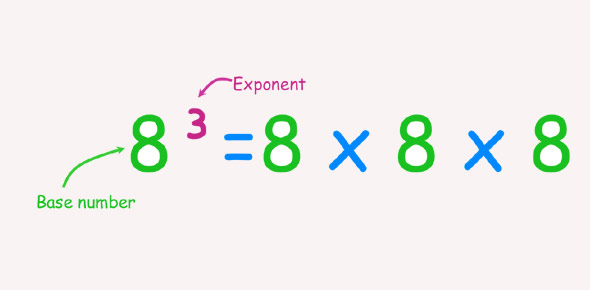Mathematics Exponents Unit 1 Exam

20 Questions | Total Attempts: 367SettingsThis test is worth 20 points. Pay attention to how exponents are written. X to the fifth power is written like x^5Fractions will be written like 1/2, meaning one-half

• 1.
Express using exponents
• A.

-5^3

• B.

-15

• C.

-1/125

• D.

5^-3

• 2.
Express using exponents.
• A.

X^4 * y^2

• B.

4x2y

• C.

Xxxxyyy

• D.

Xy^2

• 3.
• A.

X^7

• B.

X^12

• C.

7x

• D.

X^1

• 4.
• A.

30g^5

• B.

11g^5

• C.

30g^6

• D.

11g^6

• 5.
• A.

3^6

• B.

3^4

• C.

3^10

• D.

3

• 6.
• A.

3a^6

• B.

4a^6

• C.

4a^8

• D.

3a^7

• 7.
• A.

X^8

• B.

X^6

• C.

X^2

• D.

X^24

• 8.
• A.

8c^6

• B.

6c^6

• C.

2c^6

• D.

2c^5

• 9.
What number is equivalent to?
• A.

1/16

• B.

-1/16

• C.

16

• D.

-16

• 10.
• A.

1/36

• B.

-1/36

• C.

36

• D.

-36

• 11.
Convert  to standard form.
• 12.
• 13.
Write 74,000 in scientific notation
• A.

7.4 x 10^4

• B.

74 x 10^3

• C.

.74 x 10^5

• D.

7.4 x 10^5

• 14.
Write 0.000009 in scientific notation.
• A.

9 x 10^-6

• B.

9 x 10^6

• C.

0.9 x 10^5

• D.

0.9 x 10^-5

• 15.
• A.

1.48 x 10^9

• B.

14.8 x 10^9

• C.

14.8 x 10^8

• D.

1.48 x 10^7

• 16.
• A.

7 x 10^3

• B.

.7 x 10^4

• C.

7 x 10^4

• D.

.7 x 10^10

• 17.
• 18.
• 19.
is greater than 5.
• A.

True

• B.

False

• 20.
• A.

7.16 x 10^5

• B.

71.6 x 10^4

• C.

71.6 x 10^5

• D.

10.58 x 10^9

Related TopicsBack to top